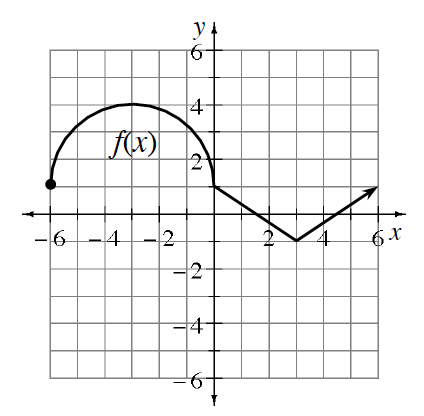### Home > INT1 > Chapter 11 > Lesson 11.2.6 > Problem11-125

11-125.

Given the graph of $f(x)$ at right, graph each transformation listed below.

1. $f(x) + 1$

1. $f(x)−3\frac{1}{3}$